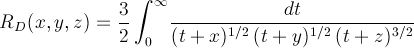Computes the Carlson integral RD.

Namespace:  Meta.Numerics.Functions
Assembly:  Meta.Numerics (in Meta.Numerics.dll) Version: 4.1.4Syntax
```public static double CarlsonD(
double x,
double y,
double z
)```

#### Parameters

x
Type: SystemDouble
The first parameter, which must be non-negative.
y
Type: SystemDouble
The second parameter, which must be non-negative.
z
Type: SystemDouble
The third parameter, which must be non-negative.

#### Return Value

Type: Double
The value of RD(x, y, z)Exceptions
ExceptionCondition
ArgumentOutOfRangeExceptionx, y, or z is negative.Remarks

The Carlson D integral is:It is symmetric with respect to the interchange of the first two parameters, but not the third parameter.

The Carlson integrals can be used to express integrals of rational functions. In that sense, they are replacements for the Legendre elliptic functions.See Also